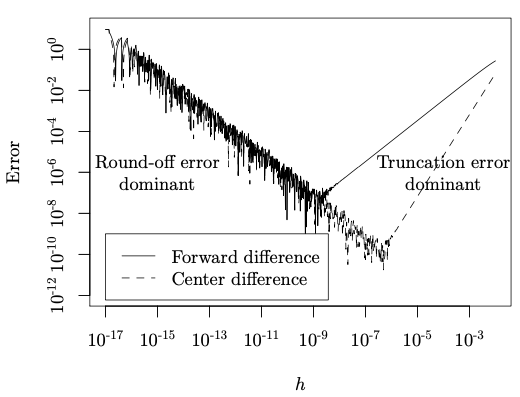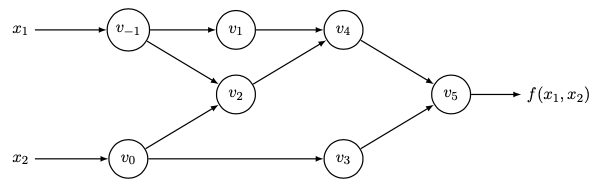2020 年 6 月 14 日

## 数值求导与符号求导

\frac{\mathrm df}{\mathrm dx_i}=\frac{f(x+h\boldsymbol{e}_i)-f(x-h\boldsymbol{e}_i)}{2h}f(x)=f_1(x)\cdots f_k(x)

## 自动求导：前向与后向

### 使用导数的运算规则来找出求导的路径，但在路径上并不展开全部的表达式，而是立即计算数值，以此避免重复计算和「表达式爆炸」的情况。\operatorname{nflops}(f_{x})\approx c\operatorname{nflops}(f)

## 计算图、求值轨迹、伴随轨迹\bar v_i=\frac{\mathrm dy}{\mathrm dv_i}

\bar v_0=\bar v_2\frac{\mathrm dv_2}{\mathrm dv_0}+\bar v_3\frac{\mathrm dv_3}{\mathrm dv_0}

\frac{\mathrm dv_2}{\mathrm v_0}=v_{-1}

## 辛可分经典系统的幂零子系统分解

\ddot x=J H_{xx}J H_{x}

x(t+\Delta t)=x(t)+\Delta t\dot x(t)

H=\sum_{i=1}^{k}a_iT+\sum_{i=1}^{k+1}b_iV

using ReverseDiff
struct System
V::Function
T::Function
V_q::Function
T_p::Function
q::Vector
p::Vector
System(V, T, q, p) = begin
new(V, T, V_q, T_p, q, p)
end
end


struct Integrator
a::Vector
b::Vector
end


(integrator!::Integrator)(system::System, dt::Real) = begin
for i in 1:length(integrator!.b)
system.p .-= integrator!.a[i] * system.V_q(system.q) * dt
system.q .+= integrator!.b[i] * system.T_p(system.p) * dt
end
system.p .-= integrator!.a[end] * system.V_q(system.q) * dt
end


awesomePotential(q) = sum(x -> .5x^2, q)
awesomeKinetic(p) = sum(x -> .5x^2, p)
system = System(awesomePotential, awesomeKinetic, rand(2), rand(2))


H=\frac12V+T+\frac12V

• p\leftarrow p-V_{q}\Delta t/2
• q\leftarrow q+T_{p}\Delta t
• p\leftarrow p-V_{q}\Delta t/2

integrator = Integrator([.5, .5], )
for _ in 1:1e5
integrator(system, .1)
end